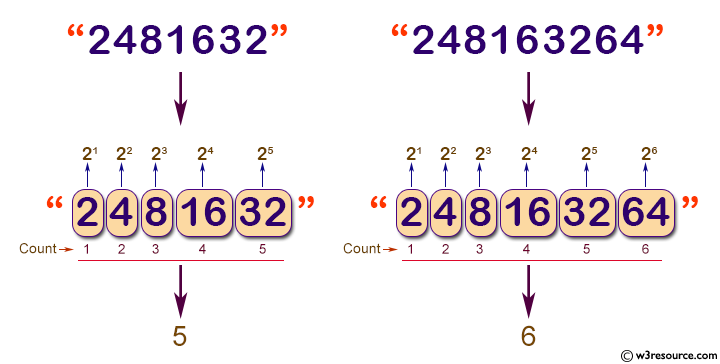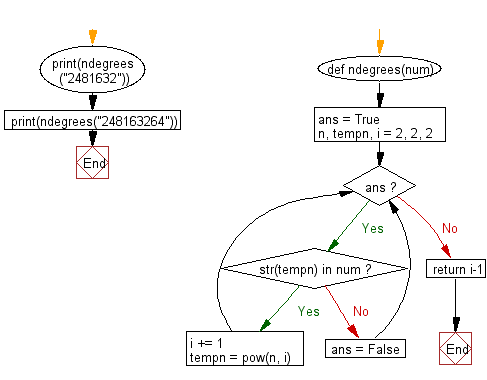﻿ Python: Find the value of n where n degrees of number 2 are written sequentially in a line without spaces - w3resource# Python: Find the value of n where n degrees of number 2 are written sequentially in a line without spaces

## Python Basic - 1: Exercise-19 with Solution

Write a Python program to find the value of n where n degrees of number 2 are written sequentially in a line without spaces.

Pictorial Presentation:Sample Solution:

Python Code:

``````def ndegrees(num):
ans = True
n, tempn, i = 2, 2, 2
while ans:
if str(tempn) in num:
i += 1
tempn = pow(n, i)
else:
ans = False
return i-1;
print(ndegrees("2481632"))
print(ndegrees("248163264"))
``````

Sample Output:

```5
6
```

Flowchart:## Visualize Python code execution:

The following tool visualize what the computer is doing step-by-step as it executes the said program:

Python Code Editor:

Have another way to solve this solution? Contribute your code (and comments) through Disqus.

What is the difficulty level of this exercise?

Test your Python skills with w3resource's quiz

﻿

## Python: Tips of the Day

Checks if the given number falls within the given range.

Example:

```def tips_range(n, start, end = 0):
return start <= n <= end if end >= start else end <= n <= start
print(tips_range(2, 4, 6))
print(tips_range(4, 8))
print(tips_range(1, 3, 5))
print(tips_range(1, 3))
```

Output:

```False
True
False
True
```